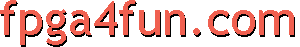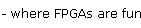## PWM DAC 2 - First-order sigma-delta modulator

A first-order sigma-delta modulator resembles a PWM, but with a better frequency response if you need to filter it because of its higher frequency output content.

The simplest way to create a first-order sigma-delta modulator is to use an hardware accumulator... every time the accumulator overflows, output a '1'. Otherwise output a '0'. That's very easily done in an FPGA.

Verilog
module PWM(clk, PWM_in, PWM_out);
input clk;
input [7:0] PWM_in;
output PWM_out;

reg [8:0] PWM_accumulator;
always @(posedge clk) PWM_accumulator <= PWM_accumulator[7:0] + PWM_in;

assign PWM_out = PWM_accumulator;
endmodule

VHDL
```library IEEE;
use IEEE.STD_LOGIC_1164.all;
use IEEE.STD_LOGIC_UNSIGNED.ALL;

entity PWM is
port (
clk : in std_logic;
PWM_in : in std_logic_vector (7 downto 0) := "00000000";
PWM_out : out std_logic
);
end PWM;

architecture PWM_arch of PWM is
signal  PWM_Accumulator : std_logic_vector(8 downto 0);
begin
process(clk, PWM_in)
begin
if rising_edge(clk) then
PWM_Accumulator  <=  ("0" & PWM_Accumulator(7 downto 0)) + ("0" & PWM_in);
end if;
end process;

PWM_out <= PWM_Accumulator(8);
end PWM_arch;
```

The higher the input value, the faster the accumulator overflows ("PWM_accumulator"), and the more frequent are the output "1"s.